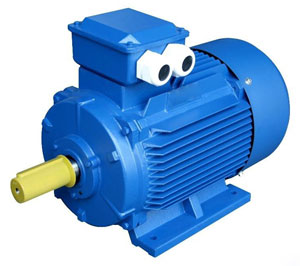Home » Three Phase ConverterOften the European motors at 1hp size are universal for 50Hz or 60Hz power supply, as long as you have 400V x 50Hz and 460V x 60Hz. This is because the voltage curve from 0V x 0Hz is much the same power line through 400 x 50Hz on its way to 460V x 60Hz, so the motor is still fluxed correctly. At 60Hz power source it will usually be capable of increased power, but you will need to refer to the manufacturer's data to confirm this, and how many poles and who is the manufacturer.

Another point touched on here, is the pump. Is this a European pump, or are you retrofitting the motor? If the motor and Pump are made for use on 50Hz power supply, then there will be much more power demanded by the load. This is because at 60Hz speed will increase by 20% and absorbed kW follows the 'Cube' of speed (given that nothing else changes).
Since Power = Torque x Speed, the same motor will be able to produce approximately twice the power at 100 Hz that it will at 50 Hz - assuming of course it can rotate safely at that speed.

It emphasizes also that it is very desirable to contact the motor manufacturer with questions like this. All of the general guidelines are correct and generally true but much depends on the motor design as stated. The manufacturer has design data making it very easy to answer this question, likely doing this every day. The difference between 50Hz and 60Hz power supply usually 20% - nominal power at 60Hz higher by 20% of 50Hz power supply. The data can be found on motor nameplate.Since the formula for governing the synchronous speed of a three-phase motor is = [(120* Hz)/# Motor Poles] if this is a 4-pole motor then at 50 Hz the speed would be 1500 RPM whereas at 60 Hz the speed would be 1800 RPM. Since motors are constant torque machines then by applying the formula that HP = (torque*RPM)/5252 then you can see that with a 20% increase in speed the motor would also then be able to produce 20% more horsepower. The motor would be able to produce rated torque at both frequencies only apply if the V/Hz ratio is constant, meaning that at 50 Hz the supply voltage would need to be 380V and at 60 Hz the supply voltage would need to be 460V. In both cases the V/Hz ratio is 7.6 V/Hz.

A potential solution for this problem would be to apply a variable frequency drive in this application which would be able to take in the 460V/60 Hz power source and output 380V/50Hz to the motor, putting everything back to the original design.
An induction motor at 0.75 kW (approx. 1 HP), 50 Hz, 400v AC, set the motor Hz in the VFD to 60, then the Motor Voltage to 480v AC. This will increase the motor output to approx. 0.9 kW, (approx. 1.2 HP) with a corresponding increase in torque. Setting the motor speed (RPM) using the 20% factor is also essential. For example, if the motor speed @ 50 Hz is 1,400 RPM, dividing the RPM total by 0.8, results in a quotient of 1,750 RPM. By affording this balance of the Volts/Hz/RPM ratio, the equipment design criteria is very closely matched in the conversion.

In the US, the power grid is not as uniform as it is in many areas of Europe and elsewhere, with voltage levels fluctuating significantly from grid to grid. These levels will vary from approx. 460 VAC to 490 VAC in most areas in Wye Delta supply. In Grounded Delta (aka 'B' Phase Delta) the line variances can spread from 440 VAC to 525 VAC, depending on the power grid source and how many facilities draw power simultaneously along that grid supply.

50Hz 60Hz Frequency Converter Setting
Using GoHz frequency converter to
• Convert 220v 50Hz to 110v 60Hz,
• Convert 120v 60Hz to 230v 50Hz,
• Convert 110v 60Hz to 240v 50Hz,
...
• Convert 480v 60Hz to 380v 50Hz,
• Convert 400v 50Hz to 460v 60Hz,
• Convert 240v 60Hz to 380v 50Hz,
...
460v 60Hz motor on 400v 50Hz power ...Often the European motors at 1hp size are universal for 50Hz or 60Hz power supply, as long as you have 400V x 50Hz and 460V x ...
Convert 220v, 230v, 240v 50Hz to 110v, ...When you buy an 110v (120v) 60Hz appliance from USA, and run it on 220v (230v, 240v) 50Hz country (i.e. UK, Australia, ...
60Hz motor running on 50Hz power ...Electric motors, both single and three phase, are designed for running on a specified power frequency. But sometimes we may use a ...